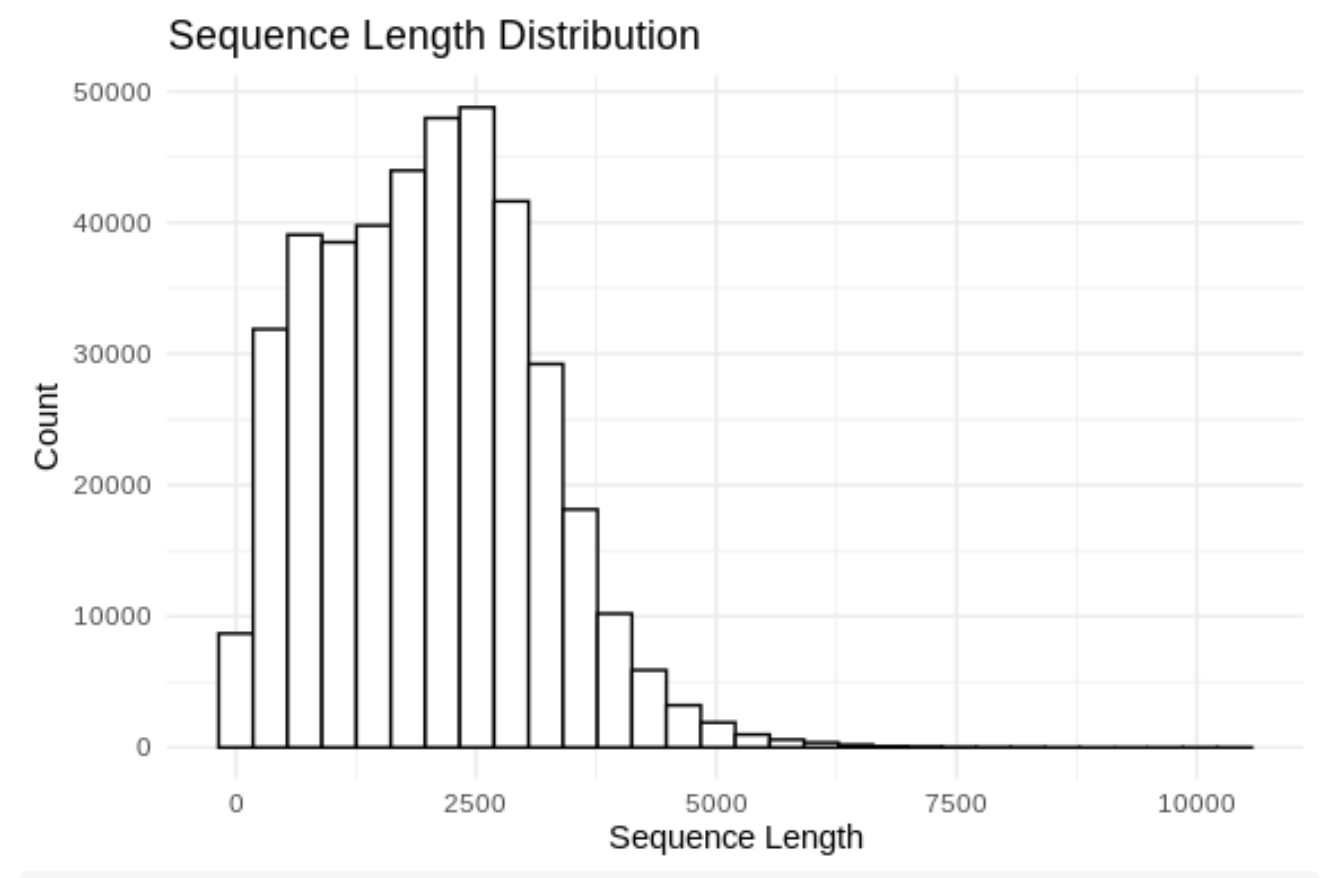# Mytilus trossulus Iso-seq

Lets take look at Isoseq fasta

``````cd ../data/

curl -O https://owl.fish.washington.edu/halfshell/genomic-databank/Mtros-hq_transcripts.fasta
``````
``````# Load the sequences from a fasta file
fasta_path <- "../data/Mtros-hq_transcripts.fasta" # Change to your FASTA file path

# Calculate the lengths of the sequences
sequence_lengths <- width(dna_sequences)

# Convert to a data frame for ggplot
df <- data.frame(Length = sequence_lengths)

# Plot the length distribution
ggplot(df, aes(x = Length)) +
geom_histogram(color = "black", fill = "white", bins = 30) +
theme_minimal() +
labs(title = "Sequence Length Distribution",
x = "Sequence Length",
y = "Count")
````````````# Number of sequences shorter than 500 bp
short_sequences <- sum(sequence_lengths < 500)
print(short_sequences)
``````
``````##  36551
``````
``````really_short_sequences <- sum(sequence_lengths < 200)
print(really_short_sequences)
``````
``````##  10038
``````
Written on July 20, 2023